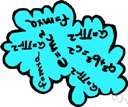# abelian group

Also found in: Thesaurus, Encyclopedia, Wikipedia.
Related to abelian group: group theory, vector space, Cyclic group

## a·be·li·an group

(ə-bē′lē-ən)
n.

[After Niels Henrik Abel (1802-1829), Norwegian mathematician.]

## Abelian group

(əˈbiːlɪən)
n
(Mathematics) a group the defined binary operation of which is commutative: if a and b are members of an Abelian group then ab = ba
[C19: named after Niels Henrik Abel (1802–29), Norwegian mathematician]
Collins English Dictionary – Complete and Unabridged, 12th Edition 2014 © HarperCollins Publishers 1991, 1994, 1998, 2000, 2003, 2006, 2007, 2009, 2011, 2014
ThesaurusAntonymsRelated WordsSynonymsLegend:
 Noun 1Abelian group - a group that satisfies the commutative lawcommutative groupmathematical group, group - a set that is closed, associative, has an identity element and every element has an inverse
Based on WordNet 3.0, Farlex clipart collection. © 2003-2012 Princeton University, Farlex Inc.
Translations
Mentioned in ?
References in periodicals archive ?
So, an element u [member of] [R.sup.x] is a unit of R if there exist u' [member of] [R.sup.x] such that uu' = u'u = 1, and [R.sup.x] forms an abelian group under usual multiplication of R.
This math text explains the origins and underlying assumptions of the Cantor set, an ordered abelian group. Chapters are devoted to the Bratteli-Vershik model, the Effros-Handelman-Shen theorem, the Bratelli-Elliott-Krieger theorem, measure theory, and the absorption theorem.
In the case that X is a commutative ring or a finite abelian group (written multiplicative) and f maps x into its k-th power in X, we denote G(X, f) by G(X, k).
Every finite Abelian group is determined by its endomorphism monoid in the class of all groups.
Let G be an abelian group and [alpha] be a fixed nonzero element of G.
Then G/G' is an abelian group and G' is minimal with respect to this property.
Let H be a finite abelian group written additively and End(H) be the endomorphism ring of H.
More precisely, for a field k, a joint determinant D (= [D.sub.l]) (l [greater than or equal to] 1) is defined as a map from the set of l-tuples of commuting matrices in [GL.sub.n](k) (n [greater than or equal to] 1) into some abelian group (G, +) which satisfies the following properties.
The infinite-dimensional torus [OMEGA] with the product topology and operation of pointwise multiplication is a compact topological Abelian group. Therefore, on ([OMEGA], b([OMEGA])), where b(X) is the Borel [sigma]-field of the space X, the probability Haar measure [m.sub.H] exists, and this gives the probability space ([OMEGA], b([OMEGA]), [m.sub.H]).
In order to study self-dual abelian codes, it is therefore restricted to the group algebra [mathematical expression not reproducible], where A is an abelian group of odd order and B is a nontrivial abelian group of two power order.

Site: Follow: Share:
Open / Close